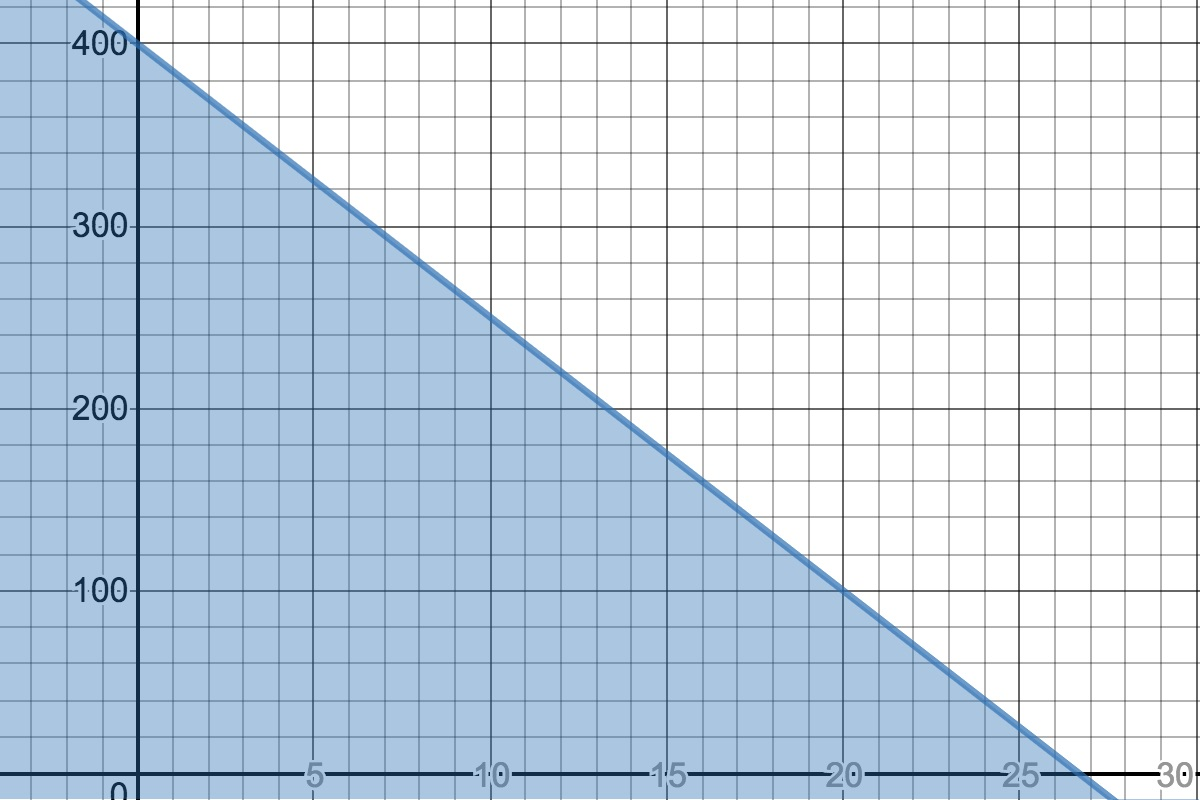# LINR 2 | Lesson 3 | Explore 1 Solution

Let’s look at the following responses about Jamal’s trip:

Rule: $y\leq 400-15x$

• If your rule is different, compare and contrast both versions. Why are they different? How can we tell which one is correct, equivalent or not.
• What does the $$x$$, $$y$$, $$400$$, $$-15$$, represent in this situation?
• Choose an $$(x, y)$$ coordinate. How can you tell if this is solution, or not?

Graph(created using Desmos):• Is the inequality a function? Why or why not?
• This graph shows values of $$x\geq 0$$ and $$y\geq 0$$. Is this reasonable in this situation?
• Enter some coordinate solutions a table. How is this different from an equality table?